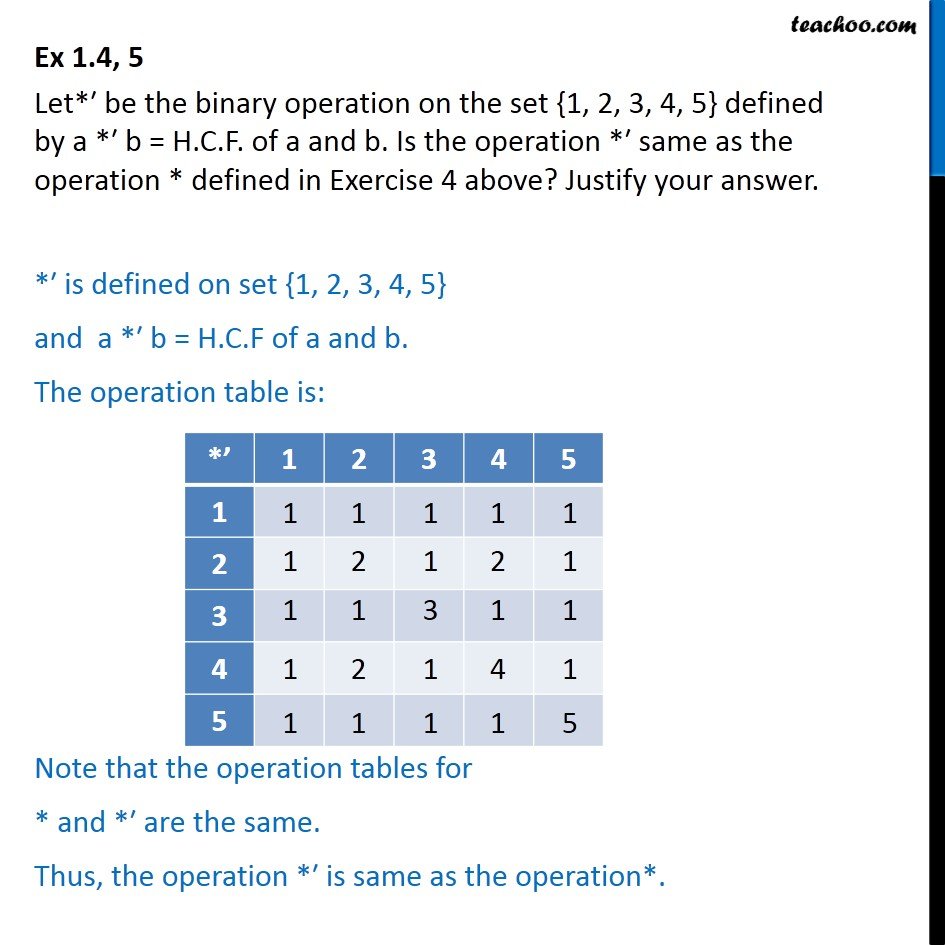1. Chapter 1 Class 12 Relation and Functions (Term 1)
2. Serial order wise
3. Ex 1.4

Transcript

Ex 1.4, 5 Let* be the binary operation on the set {1, 2, 3, 4, 5} defined by a * b = H.C.F. of a and b. Is the operation * same as the operation * defined in Exercise 4 above? Justify your answer. * is defined on set {1, 2, 3, 4, 5} and a * b = H.C.F of a and b. The operation table is: Note that the operation tables for * and * are the same. Thus, the operation * is same as the operation*.